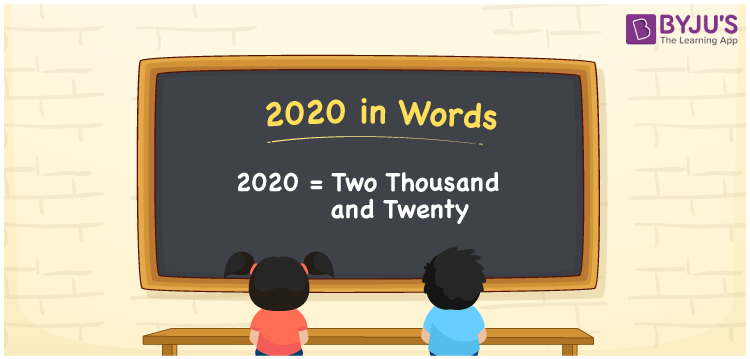# 2020 in words

2020 in words is written as Two Thousand and Twenty. 2020 represents the count or value. The article on Counting Numbers can give you an idea about count or counting. The number 2020 is used in expressions that relate to money, distance, length, year and others. Let us consider an example for 2020. “The pandemic was at a peak in the year Two Thousand and Twenty.” Another example, “The strength of all the primary classes is Two Thousand and Twenty.”

 2020 in words Two Thousand and Twenty Two Thousand and Twenty in Numbers 2020

## 2020 in English Words## How to Write 2020 in Words?

We can convert 2020 to words using a place value chart. The number 2020 has 4 digits, so let’s make a chart that shows the place value up to 4 digits.

 Thousands Hundreds Tens Ones 2 0 2 0

Thus, we can write the expanded form as:

2 × Thousand + 0 × Hundred + 2 × Ten + 0 × One

= 2 × 1000 + 0 × 100 + 2 × 10 + 0 × 1

= 2020

= Two Thousand and Twenty.

2020 is the natural number that is succeeded by 2019 and preceded by 2021.

2020 in words – Two Thousand and Twenty.

Is 2020 an odd number? – No.

Is 2020 an even number? – Yes

Is 2020 a perfect square number? – No

Is 2020 a perfect cube number? – No

Is 2020 a prime number? – No

Is 2020 a composite number? – Yes

## Solved Example

1. Write the number 2020 in expanded form

Solution: 2 × 1000 + 0 × 100 + 2 × 10 + 0 × 1

We can write 2020 = 2000 + 000 + 20 + 0

= 2 × 1000 + 0 × 100 + 2 × 10 + 0 × 1

## Frequently Asked Questions on 2020 in words

Q1

### How to write 2020 in words?

2020 in words is written as Two Thousand and Twenty.
Q2

### Is 2020 divisible by 2?

Yes. 2020 is divisible by 2.
Q3

### Is 2020 divisible by 10?

Yes. 2020 is divisible by 10.